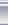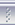# 岁月流转，往昔空明C++博客首页新随笔联系聚合管理#### 入口函数与非入口函数

```float4x4 wvpMat;

struct VS_INPUT{
float4 pos: SV_Position;
float4 tex: SV_Texcoord0;
};

struct VS_OUTPUT{
float4 pos: SV_Position;
float4 tex: SV_Texcoord0;
};

float4 world_pos( float4 p ){
return mul(p, wvpMat);
}

VS_OUTPUT vs_main(VS_INPUT in){
VS_OUTPUT o;
o.pos = world_pos(in.pos);
o.tex = in.tex;
return o;
}
```

#### 数据结构与入口签名

SASL最终将生成如下的签名：

```struct stream_in{
float4* pos;
float4* tex;
};

struct buffer_in{ float4x4 wvpMat; };
struct stream_out{}; // empty.
struct buffer_out{
float4 pos;
float4 tex;
};

float4 world_pos( float4 pos, buffer_in* bi );
void vs_main( stream_in* si, buffer_in* bi, stream_out* so, buffer_out* bo );
```

#### 结构体的语义布局与常规布局

```void vs_main( stream_in* si, buffer_in* bi, stream_out* so, buffer_out* bo ){
// initialization
VS_INPUT __tmp_in = {*si->pos, *si->tex};
VS_OUTPUT __tmp_out;
// end initialization

VS_OUTPUT o;
o.pos = world_pos( __tmp_in.pos, bi );
o.tex = __tmp_in.tex;

__tmp_out = o;

// return
bo->pos = __tmp_out.pos;
bo->tex = __tmp_out.tex;
return;
// end return
}
```

posted on 2011-04-14 10:23 空明流转 阅读(1495) 评论(0)  编辑 收藏 引用